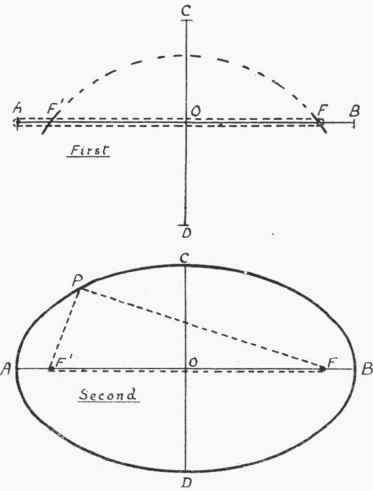There are many objects of elliptical shape that require to be made up out of sheet and plate metal. It is, therefore, essential that workmen in these trades should know one or two practical methods for describing ellipses. Whilst there are a great number of different ways in which an ellipse may be set out, there are only two that are of much use for workshop purposes. We shall now proceed to describe these two methods.

Construction Of Ellipse

One way of describing the ellipse is that known as the "string method," and is illustrated by Fig. 165. The diameters A B and C D are first set out at right angles, as there shown, and the points F', F (called the foci) obtained by setting the compasses at half the long diameter and using C or D as centre, and cutting A B in F', F. A pin or nail is now stuck in each of the points A and F, and a piece of string brought round the two nails, as shown, and tied. The nail is then drawn out of A and fixed in F', as seen in the lower figure. The string is stretched tight by holding a scriber or pencil, as at P, and at the same time the ellipse described by moving the pencil all round, as shown. To get an accurate result, string that has very little stretch should be used.

If it is desired to mark an ellipse on a plate or sheet, where there will be difficulty in fixing the pins, a good plan is to clamp a batten on to the plate and drive the pins into this.

There is an important property of the ellipse which is worth while remembering, and that is: "The sum of the distances of the foci from any point on the ellipse is a constant quantity, and is equal to the long diameter." It is, indeed, from this property that we are enabled to construct the ellipse by the string method; for if the lengths P F', P F be added together, they will, for any position of P, be equal to A B. Knowing this peculiarity of the ellipse, the ingenious reader should be enabled to devise one or two other simple methods for its construction.Fig. 165.

The string method is most adaptable for large ellipses, and for smaller ones what is known as the "trammel method" is most suitable. This latter will now be explained,

A trammel (Fig. 166) need be nothing more than a strip of cardboard, wood, or sheet metal. Half the long diameter, P E, and half the short diameter, P F, of the ellipse must first be set along from the end of the trammel. And then to construct the ellipse, two lines at right angles are drawn, and on these the trammel placed, the points e f being respectively on the lines D C and A B. The trammel is now moved into different positions, points on the ellipse being obtained by marking the end P. When a sufficient number of points are obtained they can be connected with an even curve, and so the whole ellipse described. The important thing to notice is that the point e must always slide on the line D C at the same time as point f is moving along line A B. Two positions of the trammel are shown on Fig. 166.Fig. 166.

It is not a difficult matter to make a trammel with two adjustable pegs and wood or metal cross-shaped slides (to lie along the ellipse diameters), and with this simple apparatus construct ellipses in a similar manner to that in which circles are described by compasses.

Circumference of Ellipse. ,

The circumference of an ellipse can be found by adding together the semi-diameters and multiplying by 3 1/7 Thus in Fig. 165, suppose A B = 24 in. and CD = 18 in.; then the circumference equals -

(12 + 9) x 3 1/7 = 21 X 22 / 7 = 66 m.

The rule, as given, is only approximately correct, but if good enough for workshop practice when the ellipse is not very flat. Calculated by a rule giving more accurate results, the circumference should be about § in. longer than above. Unfortunately, however, very accurate results are difficult in manipulation. In practice, the simplest way of obtaining the length of the circumference is to bend a thin wire along a quarter of the ellipse, as set out, and multiply this length by 4.